CGAL 5.3 - 3D Triangulations
User Manual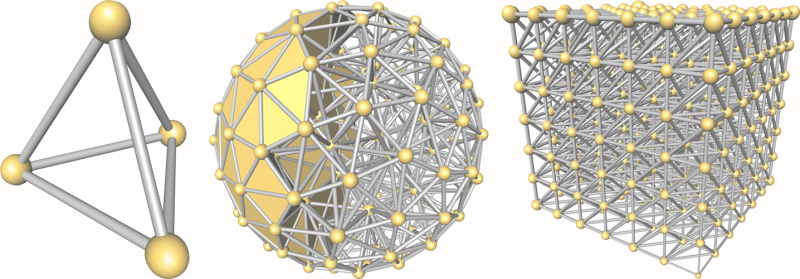The basic 3D-triangulation class of CGAL is primarily designed to represent the triangulations of a set of points $$A$$ in $$\mathbb{R}^3$$. It is a partition of the convex hull of $$A$$ into tetrahedra whose vertices are the points of $$A$$. Together with the unbounded cell having the convex hull boundary as its frontier, the triangulation forms a partition of $$\mathbb{R}^3$$. Its cells ( $$3$$-faces) are such that two cells either do not intersect or share a common facet ( $$2$$-face), edge ( $$1$$-face) or vertex ( $$0$$-face).

# Representation

In order to deal only with tetrahedra, which is convenient for many applications, the unbounded cell can be subdivided into tetrahedra by considering that each convex hull facet is incident to an infinite cell having as fourth vertex an auxiliary vertex called the infinite vertex. In that way, each facet is incident to exactly two cells and special cases at the boundary of the convex hull are simple to deal with.

The class Triangulation_3<TriangulationTraits_3,TriangulationDataStructure_3> of CGAL implements this point of view and therefore considers the triangulation of the set of points as a set of finite and infinite tetrahedra. Notice that the infinite vertex has no significant coordinates and that no geometric predicate can be applied on it.

A triangulation is a collection of vertices and cells that are linked together through incidence and adjacency relations. Each cell gives access to its four incident vertices and to its four adjacent cells. Each vertex gives access to one of its incident cells.

The four vertices of a cell are indexed with 0, 1, 2 and 3 in positive orientation, the positive orientation being defined by the orientation of the underlying Euclidean space $$\mathbb{R}^3$$ (see Figure 45.1). The neighbors of a cell are also indexed with 0, 1, 2, 3 in such a way that the neighbor indexed by $$i$$ is opposite to the vertex with the same index.
Figure 45.1 Orientation of a cell (3-dimensional case).

As in the underlying combinatorial triangulation (see Chapter 3D Triangulation Data Structure), edges ( $$1$$-faces) and facets ( $$2$$-faces) are not explicitly represented: a facet is given by a cell and an index (the facet i of a cell c is the facet of c that is opposite to the vertex with index i) and an edge is given by a cell and two indices (the edge (i,j) of a cell c is the edge whose endpoints are the vertices of c with indices i and j). See Figure 46.1.

Degenerate Dimensions

The class Triangulation_3 can also deal with triangulations whose dimension $$d$$ is less than 3. A triangulation of a set of points in $$\mathbb{R}^d$$ covers the whole space $$\mathbb{R}^d$$ and consists of cells having $$d+1$$ vertices: some of them are infinite, they are obtained by linking the additional infinite vertex to each facet of the convex hull of the points.

• dimension 2: when a triangulation only contains coplanar points (which is the case when there are only three points), it consists of triangular faces.
• dimension 1: the triangulation contains only collinear points (which is the case when there are only two points), it consists of edges.
• dimension 0: the triangulation contains only one finite point.
• dimension -1: this is a convention to handle the case when the only vertex of the triangulation is the infinite one.

The same cell class is used in all cases: triangular faces in 2D can be considered as degenerate cells, having only three vertices (resp. neighbors) numbered $$(0,1,2)$$; edges in 1D have only two vertices (resp. neighbors) numbered $$0$$ and $$1$$.

The implicit representation of facets (resp. edges) still holds for degenerate dimensions (i.e. dimensions $$<3$$): in dimension 2, each cell has only one facet of index 3, and 3 edges $$(0,1)$$, $$(1,2)$$ and $$(2,0)$$; in dimension 1, each cell has one edge $$(0,1)$$.

Validity

A triangulation of $$\mathbb{R}^3$$ is said to be locally valid iff

(a)-(b) Its underlying combinatorial graph, the triangulation data structure, is locally valid (see Section Introduction of Chapter 3D Triangulation Data Structure)

(c) Any cell has its vertices ordered according to positive orientation. See Figure 45.1.

When the triangulation is degenerated into a triangulation of dimension 2, the geometric validity reduces to:

(c-2D) For any two adjacent triangles $$(u,v,w_1)$$ and $$(u,v,w_2)$$ with common edge $$(u,v)$$, $$w_1$$ and $$w_2$$ lie on opposite sides of $$(u,v)$$ in the plane.

When all the points are collinear, this condition becomes:

(c-1D) For any two adjacent edges $$(u,v)$$ and $$(v,w)$$, $$u$$ and $$w$$ lie on opposite sides of the common vertex $$v$$ on the line.

The method Triangulation_3::is_valid() checks the local validity of a given triangulation. This does not always ensure global validity ,  but it is sufficient for practical cases.

# Delaunay Triangulation

The class Delaunay_triangulation_3 represents a three-dimensional Delaunay triangulation.

Delaunay triangulations have the specific empty sphere property, that is, the circumscribing sphere of each cell of such a triangulation does not contain any other vertex of the triangulation in its interior. These triangulations are uniquely defined except in degenerate cases where five points are co-spherical. Note however that the CGAL implementation computes a unique triangulation even in these cases.

This implementation is fully dynamic: it supports insertions of points, vertex removals and displacements of points.

# Regular Triangulation

The class Regular_triangulation_3 implements incremental regular triangulations, also known as weighted Delaunay triangulations.

Let $${p}^{(w)}=(p,w_p), p\in\mathbb{R}^3, w_p\in\mathbb{R}$$ and $${z}^{(w)}=(z,w_z), z\in\mathbb{R}^3, w_z\in\mathbb{R}$$ be two weighted points. A weighted point $${p}^{(w)}=(p,w_p)$$ can also be seen as a sphere of center $$p$$ and radius $$\sqrt{w_p}$$. The power product between $${p}^{(w)}$$ and $${z}^{(w)}$$ is defined as

$\Pi({p}^{(w)},{z}^{(w)}) = {\|{p-z}\|^2-w_p-w_z}$

where $$\|{p-z}\|$$ is the Euclidean distance between $$p$$ and $$z$$.

The weighted points $${p}^{(w)}$$ and $${z}^{(w)}$$ are said to be orthogonal iff $$\Pi{({p}^{(w)},{z}^{(w)})} = 0$$ (see Figure 45.2).

Figure 45.2 Orthogonal weighted points (picture in 2D).

Four weighted points have a unique common orthogonal weighted point called the power sphere. The weighted point orthogonal to three weighted points in the plane defined by these three points is called the power circle. The power segment will denote the weighted point orthogonal to two weighted points on the line defined by these two points.

Let $${S}^{(w)}$$ be a set of weighted points in $$\mathbb{R}^3$$. A sphere $${z}^{(w)}$$ is said to be regular if $$\forall {p}^{(w)}\in{S}^{(w)}, \Pi{({p}^{(w)},{z}^{(w)})}\geq 0$$.

A triangulation of $${S}^{(w)}$$ is regular if the power spheres of all simplices are regular.

The regular triangulation of $${S}^{(w)}$$ is in fact the projection onto $$\mathbb{R}^3$$ of the convex hull of the four-dimensional points $$(p,\|p-O\|^2-w_p),$$ for $${p}^{(w)}=(p,w_p)\in{S}^{(w)}$$. Note that all points of $${S}^{(w)}$$ do not necessarily appear as vertices of the regular triangulation. To know more about regular triangulations, see for example .

When all weights are 0, power spheres are nothing more than circumscribing spheres, and the regular triangulation is exactly the Delaunay triangulation.

The implementation of 3D regular triangulation supports insertions of weighted points, and vertex removals. Displacements are not supported in the current implementation.

# Software Design

The main classes Triangulation_3, Delaunay_triangulation_3 and Regular_triangulation_3 are connected to each other by the derivation diagram shown in Figure 45.3. This diagram also shows another class: Triangulation_utils_3, which provides a set of tools operating on the indices of vertices in cells.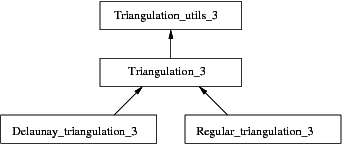Figure 45.3 Derivation diagram of the 3D triangulation classes.

The three main classes (Triangulation_3, Delaunay_triangulation_3 and Regular_triangulation_3) provide high-level geometric functionality such as location of a point in the triangulation , insertion and possibly removal of a point , and are responsible for the geometric validity. They are built as layers on top of a triangulation data structure, which stores their combinatorial structure. This separation between the geometry and the combinatorics is reflected in the software design by the fact that these three triangulation classes take the following template parameters :

• the geometric traits class, which provides the type of points to use as well as the elementary operations on them (predicates and constructions). The concepts for these parameters are described in more details in Section The Geometric Traits Parameter.
• the triangulation data structure class, which stores their combinatorial structure, described in Section Software Design of Chapter 3D Triangulation Data Structure.
• the location policy tag, which is supported only by the Delaunay triangulation class, described in Section The Location Policy Parameter.

Optionally, the main Delaunay and regular triangulations algorithms (insert, remove) support multi-core shared-memory architectures to take advantage of available parallelism. For this purpose, a model of the concept SurjectiveLockDataStructure can be given as fourth template parameter; it defaults to Spatial_lock_grid_3. This data structure allows to lock points with coordinates (x, y, z) in a 3D domain. When a thread owns the lock on a point, no other thread can lock this point. Locking a facet (resp. a cell) boils down to locking all its 3 (resp. 4) incident vertices. See Section Parallel Algorithms for more details.

## The Geometric Traits Parameter

The first template parameter of the triangulation class Triangulation_3<TriangulationTraits_3, TriangulationDataStructure_3> is the geometric traits class, described by the concept TriangulationTraits_3. It must define the types of the geometric objects (points, segments, triangles and tetrahedra) forming the triangulation together with a few geometric predicates on these objects: orientation in space, orientation in case of coplanar points, order of collinear points.

In addition to the requirements described before, the geometric traits class of Delaunay_triangulation_3 must define predicates to test for the empty sphere property. It is described by the concept DelaunayTriangulationTraits_3, which refines TriangulationTraits_3.

In addition to the requirements described before, the geometric traits class of Regular_triangulation_3 must define predicates to test for the power distances and orientation tests for power spheres. It is described by the concept RegularTriangulationTraits_3, which refines TriangulationTraits_3.

All kernels provided by CGAL can all be used as models for the geometric traits parameter.

## The Triangulation Data Structure Parameter

The second template parameter of the main classes (Triangulation_3, Delaunay_triangulation_3 and Regular_triangulation_3) is a triangulation data structure class. This class can be seen as a container for the cells and vertices maintaining incidence and adjacency relations (see Chapter chapterTDS3). A model of this triangulation data structure is Triangulation_data_structure_3, and it is described by the TriangulationDataStructure_3 concept . This model is itself parameterized by a vertex base and a cell base classes, which gives the possibility to customize the vertices and cells used by the triangulation data structure, and hence by the geometric triangulation using it. Depending on the kind of triangulation used, the requirements on the vertex and cell base classes vary, and are expressed by various concepts, following the refinement diagram shown in Figure 45.4.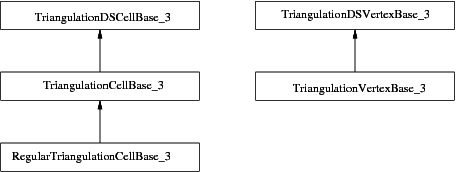Figure 45.4 Concepts refinement hierarchy for the vertex and cell base classes parameters.

A default value for the triangulation data structure parameter is provided in all the triangulation classes, so it need not be specified by the user unless he wants to use a different triangulation data structure or a different vertex or cell base class.

## The Location Policy Parameter

The Delaunay triangulation class supports an optional feature which maintains an additional data structure for fast point location queries. The fast location policy should be used when the user inserts points in a random order or needs to do many unrelated queries. If the user is able to give a good hint to help the point location of its queries (and its newly inserted points), then it should prefer the default policy. In such a case where good hints are provided, the default policy save some memory (few percents), and is faster. Notice that if points are not inserted one by one, but as a range, then a good hint is automatically computed using spatial sort.

Reading Section Complexity and Performance on complexity and performance can help making an informed choice for this parameter.

The point location strategy can be selected with the third template argument of Delaunay_triangulation_3, LocationPolicy, which enables a fast point location data structure when set to Fast_location. By default, it uses Compact_location.

Note that you can specify the LocationPolicy parameter without specifying the triangulation data structure, in case you are fine with the default there. In this case, the LocationPolicy appears as a second parameter after the geometric traits.The mechanism used behind the scenes to allow this syntactical convenience is called deduced parameters.

The Fast_location policy is implemented using a hierarchy of triangulations; it changes the behavior of functions locate, insert, move, and remove. As proved in , this structure has an optimal behavior when it is built for Delaunay triangulations.

In this setting, if you build a triangulation by iteratively inserting points, you should try to shuffle the points beforehand, as the time complexity is guaranteed only for a randomized order. For example, inserting points in lexicographic order is typically much slower. Note that this shuffling is performed internally by the constructor taking a range of points.

Prior to CGAL 3.6, this functionality was available through the Triangulation_hierarchy_3 class, which is now deprecated.

## Flexibility of the Design

In order to satisfy as many uses as possible, a design has been selected that allows to exchange different parts to meet the users' needs, while still re-using a maximum of the provided functionalities. We have already seen that the main triangulation classes are parameterized by a geometric traits class and a triangulation data structure (TDS), so that each of them can be interchanged with alternate implementations.

The most useful flexibility is the ability given to the user to add his own data in the vertices and cells by providing his own vertex and cell base classes to Triangulation_data_structure_3. The Figure 45.5 shows in more detail the flexibility that is provided, and the place where the user can insert his own vertex and/or cell base classes.
Figure 45.5 Triangulation software design.

The design of the triangulation data structure gives the possibility to store any kind of data, including handles (an entity akin to pointers) directly in the vertex and cell base classes.

To do so, there are three possibilities. The simplest one is to use the class Triangulation_vertex_base_with_info_3, and this approach is illustrated in a following subsection Adding a Color. The most complicated one, and probably useless for almost all cases, is to write a vertex base class from scratch, following the documented requirements. This is mostly useless because most of the time it is enough to derive from the models that CGAL provides, and add the desired features. In this case, when the user needs to access some type that depends on the triangulation data structure (typically handles), then he should write something like:

...
template < class GT, class Vb = Triangulation_vertex_base<GT> >
class My_vertex
: public Vb
{
public:
typedef typename Vb::Point Point;
typedef typename Vb::Cell_handle Cell_handle;
template < class TDS2 >
struct Rebind_TDS {
typedef typename Vb::template Rebind_TDS<TDS2>::Other Vb2;
typedef My_vertex<GT, Vb2> Other;
};
My_vertex() {}
My_vertex(const Point&p) : Vb(p) {}
My_vertex(const Point&p, Cell_handle c) : Vb(p, c) {}
...
};
... // The rest has not changed

The situation is exactly similar for cell base classes. Section Software Design provides more detailed information.

## Parallel Algorithms

Parallel algorithms of Delaunay_triangulation_3 and Regular_triangulation_3 are enabled if the TriangulationDataStructure_3::Concurrency_tag type is Parallel_tag and a reference to a lock data structure instance is provided via the constructor or by using Triangulation_3::set_lock_data_structure. This data structure must be a model of the concept SurjectiveLockDataStructure and can be optionally given as a template parameter of the triangulation; it defaults to Spatial_lock_grid_3.

Note that the parallel Delaunay triangulation must use the default compact location policy (and not the fast one). If those conditions are fulfilled, the insertion/removal of a range of points will be performed in parallel, and the individual insert/remove operations will be optionally thread-safe.

Parallel algorithms require the program to be linked against the Intel TBB library. To control the number of threads used, the user may use the tbb::task_scheduler_init class. See the TBB documentation for more details.

# Examples

## Basic Example

This example shows the incremental construction of a 3D triangulation, the location of a point and how to perform elementary operations on indices in a cell. It uses the default parameter of the Triangulation_3 class.

#include <CGAL/Exact_predicates_inexact_constructions_kernel.h>
#include <CGAL/Triangulation_3.h>
#include <iostream>
#include <fstream>
#include <cassert>
#include <list>
#include <vector>
typedef CGAL::Triangulation_3<K> Triangulation;
typedef Triangulation::Cell_handle Cell_handle;
typedef Triangulation::Vertex_handle Vertex_handle;
typedef Triangulation::Locate_type Locate_type;
typedef Triangulation::Point Point;
int main()
{
// construction from a list of points :
std::list<Point> L;
L.push_front(Point(0,0,0));
L.push_front(Point(1,0,0));
L.push_front(Point(0,1,0));
Triangulation T(L.begin(), L.end());
Triangulation::size_type n = T.number_of_vertices();
// insertion from a vector :
std::vector<Point> V(3);
V = Point(0,0,1);
V = Point(1,1,1);
V = Point(2,2,2);
n = n + T.insert(V.begin(), V.end());
assert( n == 6 ); // 6 points have been inserted
assert( T.is_valid() ); // checking validity of T
Locate_type lt;
int li, lj;
Point p(0,0,0);
Cell_handle c = T.locate(p, lt, li, lj);
// p is the vertex of c of index li :
assert( lt == Triangulation::VERTEX );
assert( c->vertex(li)->point() == p );
Vertex_handle v = c->vertex( (li+1)&3 );
// v is another vertex of c
Cell_handle nc = c->neighbor(li);
// nc = neighbor of c opposite to the vertex associated with p
// nc must have vertex v :
int nli;
assert( nc->has_vertex( v, nli ) );
// nli is the index of v in nc
std::ofstream oFileT("output",std::ios::out);
// writing file output;
oFileT << T;
Triangulation T1;
std::ifstream iFileT("output",std::ios::in);
iFileT >> T1;
assert( T1.is_valid() );
assert( T1.number_of_vertices() == T.number_of_vertices() );
assert( T1.number_of_cells() == T.number_of_cells() );
return 0;
}

## Changing the Vertex Base

The following two examples show how the user can plug his own vertex base in a triangulation. Changing the cell base is similar.

When the user doesn't need to add a type in a vertex which depends on the TriangulationDataStructure_3 (e.g. a Vertex_handle or Cell_handle), then he can use the Triangulation_vertex_base_with_info_3 class to add his own information easily in the vertices. The example below shows how to add a Color this way.

#include <CGAL/Exact_predicates_inexact_constructions_kernel.h>
#include <CGAL/Delaunay_triangulation_3.h>
#include <CGAL/Delaunay_triangulation_cell_base_3.h>
#include <CGAL/Triangulation_vertex_base_with_info_3.h>
#include <CGAL/IO/Color.h>
typedef Delaunay::Point Point;
int main()
{
Delaunay T;
T.insert(Point(0,0,0));
T.insert(Point(1,0,0));
T.insert(Point(0,1,0));
T.insert(Point(0,0,1));
T.insert(Point(2,2,2));
T.insert(Point(-1,0,1));
// Set the color of finite vertices of degree 6 to red.
Delaunay::Finite_vertices_iterator vit;
for (Delaunay::Vertex_handle v : T.finite_vertex_handles())
if (T.degree(v) == 6)
v->info() = CGAL::IO::red();
return 0;
}

When the user needs to add a type in a vertex which depends on the TriangulationDataStructure_3 (e.g. a Vertex_handle or Cell_handle), then he has to derive his own vertex base class, as the following example shows.

#include <CGAL/Exact_predicates_inexact_constructions_kernel.h>
#include <CGAL/Delaunay_triangulation_3.h>
#include <CGAL/Delaunay_triangulation_cell_base_3.h>
#include <CGAL/Triangulation_vertex_base_3.h>
template < class GT, class Vb = CGAL::Triangulation_vertex_base_3<GT> >
class My_vertex_base
: public Vb
{
public:
typedef typename Vb::Vertex_handle Vertex_handle;
typedef typename Vb::Cell_handle Cell_handle;
typedef typename Vb::Point Point;
template < class TDS2 >
struct Rebind_TDS {
typedef typename Vb::template Rebind_TDS<TDS2>::Other Vb2;
typedef My_vertex_base<GT, Vb2> Other;
};
My_vertex_base() {}
My_vertex_base(const Point& p) : Vb(p) {}
My_vertex_base(const Point& p, Cell_handle c) : Vb(p, c) {}
Vertex_handle vh;
Cell_handle ch;
};
typedef Delaunay::Vertex_handle Vertex_handle;
typedef Delaunay::Point Point;
int main()
{
Delaunay T;
Vertex_handle v0 = T.insert(Point(0,0,0));
Vertex_handle v1 = T.insert(Point(1,0,0));
Vertex_handle v2 = T.insert(Point(0,1,0));
Vertex_handle v3 = T.insert(Point(0,0,1));
Vertex_handle v4 = T.insert(Point(2,2,2));
Vertex_handle v5 = T.insert(Point(-1,0,1));
// Now we can link the vertices as we like.
v0->vh = v1;
v1->vh = v2;
v2->vh = v3;
v3->vh = v4;
v4->vh = v5;
v5->vh = v0;
return 0;
}

## Setting Information While Inserting a Range of Points

The most efficient method to insert (weighted) points in a Delaunay (or regular) triangulation is to provide an iterator range over (weighted) points to the insert function. However, an iterator range of (weighted) points does not allow to set different information to each vertex. To solve this problem, in the case the vertex type of the triangulation is a model of the concept TriangulationVertexBaseWithInfo_3 (such as Triangulation_vertex_base_with_info_3), we provide three examples doing the same operation: set an unsigned integer as the information of each vertex. The value of this unsigned integer is the initial order of the corresponding point given in the range.

### Using an Iterator Over Pairs

Each point and its information are gathered into a pair. We provide the constructor of the triangulation (which is calling the insert function) with a range of such pairs.
File Triangulation_3/info_insert_with_pair_iterator.cpp

#include <CGAL/Exact_predicates_inexact_constructions_kernel.h>
#include <CGAL/Delaunay_triangulation_3.h>
#include <CGAL/Delaunay_triangulation_cell_base_3.h>
#include <CGAL/Triangulation_vertex_base_with_info_3.h>
#include <vector>
//Use the Fast_location tag. Default or Compact_location works too.
typedef Delaunay::Point Point;
int main()
{
std::vector< std::pair<Point,unsigned> > points;
points.push_back( std::make_pair(Point(0,0,0),0) );
points.push_back( std::make_pair(Point(1,0,0),1) );
points.push_back( std::make_pair(Point(0,1,0),2) );
points.push_back( std::make_pair(Point(0,0,1),3) );
points.push_back( std::make_pair(Point(2,2,2),4) );
points.push_back( std::make_pair(Point(-1,0,1),5) );
Delaunay T( points.begin(),points.end() );
CGAL_assertion( T.number_of_vertices() == 6 );
// check that the info was correctly set.
for (Delaunay::Vertex_handle v : T.finite_vertex_handles())
if( points[ v->info() ].first != v->point() ){
std::cerr << "Error different info" << std::endl;
exit(EXIT_FAILURE);
}
std::cout << "OK" << std::endl;
return 0;
}

### Using the Boost Zip Iterator

Information and points are in separate containers. We use boost::zip_iterator to provide an iterator gathering them.

#include <CGAL/Exact_predicates_inexact_constructions_kernel.h>
#include <CGAL/Delaunay_triangulation_3.h>
#include <CGAL/Delaunay_triangulation_cell_base_3.h>
#include <CGAL/Triangulation_vertex_base_with_info_3.h>
#include <boost/iterator/zip_iterator.hpp>
#include <iostream>
#include <vector>
typedef Delaunay::Point Point;
int main()
{
std::vector<unsigned> indices;
indices.push_back(0);
indices.push_back(1);
indices.push_back(2);
indices.push_back(3);
indices.push_back(4);
indices.push_back(5);
std::vector<Point> points;
points.push_back(Point(0,0,0));
points.push_back(Point(1,0,0));
points.push_back(Point(0,1,0));
points.push_back(Point(0,0,1));
points.push_back(Point(2,2,2));
points.push_back(Point(-1,0,1));
Delaunay T( boost::make_zip_iterator(boost::make_tuple( points.begin(),indices.begin() )),
boost::make_zip_iterator(boost::make_tuple( points.end(),indices.end() ) ) );
CGAL_assertion( T.number_of_vertices() == 6 );
// check that the info was correctly set.
for (Delaunay::Vertex_handle v : T.finite_vertex_handles() )
if( points[ v->info() ] != v->point() ){
std::cerr << "Error different info" << std::endl;
exit(EXIT_FAILURE);
}
return 0;
}

### Using the Boost Transform Iterator

We define a functor Auto_count used together with boost::transform_iterator to set the order of each point in the range. Note that this is correct because the iterator is dereferenced only once per point during the insertion.
File Triangulation_3/info_insert_with_transform_iterator.cpp

#include <CGAL/Exact_predicates_inexact_constructions_kernel.h>
#include <CGAL/Delaunay_triangulation_3.h>
#include <CGAL/Delaunay_triangulation_cell_base_3.h>
#include <CGAL/Triangulation_vertex_base_with_info_3.h>
#include <CGAL/boost/iterator/transform_iterator.hpp>
#include <vector>
typedef Delaunay::Point Point;
//a functor that returns a std::pair<Point,unsigned>.
//the unsigned integer is incremented at each call to
//operator()
struct Auto_count : public CGAL::cpp98::unary_function<const Point&,std::pair<Point,unsigned> >{
mutable unsigned i;
Auto_count() : i(0){}
std::pair<Point,unsigned> operator()(const Point& p) const {
return std::make_pair(p,i++);
}
};
int main()
{
std::vector<Point> points;
points.push_back(Point(0,0,0));
points.push_back(Point(1,0,0));
points.push_back(Point(0,1,0));
points.push_back(Point(0,0,1));
points.push_back(Point(2,2,2));
points.push_back(Point(-1,0,1));
Delaunay T( boost::make_transform_iterator(points.begin(),Auto_count()),
boost::make_transform_iterator(points.end(), Auto_count() ) );
CGAL_assertion( T.number_of_vertices() == 6 );
// check that the info was correctly set.
for (Delaunay::Vertex_handle v : T.finite_vertex_handles())
if( points[ v->info() ] != v->point() ){
std::cerr << "Error different info" << std::endl;
exit(EXIT_FAILURE);
}
std::cout << "OK" << std::endl;
return 0;
}

## Iterators and Ranges

The triangulation defines iterator types such as Triangulation_3::All_vertices_iterator. They behave like a Triangulation_3::Vertex_handle, in the sense that there is no need to dereference the iterator to obtain a handle. Wherever the API expects a handle the iterator can be passed as well.

In order to write a C++ 11 for-loop the triangulation calls also offers the range type Triangulation_3::All_vertex_handles, which has a nested type Triangulation_3::All_vertex_handles::iterator. The value type of this iterator is Triangulation_3::Vertex_handle. It is similar for the various iterators for vertices and cells.

For the range Triangulation_3::All_facets it holds that Triangulation_3::All_facets::iterator == Triangulation_3::All_facets_iterator. It is similar for the iterators for facets, edges and points.

Note that you only need the iterator type if you combine the pre C++ 11 for-loop with the range class.

#include <CGAL/Exact_predicates_inexact_constructions_kernel.h>
#include <CGAL/Triangulation_3.h>
#include <iostream>
#include <vector>
typedef CGAL::Triangulation_3<K> Triangulation;
typedef Triangulation::Vertex_handle Vertex_handle;
typedef Triangulation::Point Point;
typedef Triangulation::Finite_vertex_handles Finite_vertex_handles;
// The following types are different
// Its value type is Triangulation_3::Vertex
typedef Triangulation::Finite_vertices_iterator Finite_vertices_iterator;
// Its value type is Triangulation_3::Vertex_handle
typedef Finite_vertex_handles::iterator Finite_vertex_handles_iterator;
int main()
{
std::vector<Point> points = { Point(0,0,0), Point(1,0,0), Point(0,1,0), Point(0,1,1) };
Triangulation T(points.begin(), points.end());
std::cout << "Triangulation_3::Finite_vertices_iterator is like a Triangulation_3::Vertex_handle\n";
for(Finite_vertices_iterator it = T.finite_vertices_begin();
it != T.finite_vertices_end();
++it){
std::cout << it->point() << std::endl;
}
std::cout << "Triangulation_3::Finite_vertex_handles::iterator dereferences to Triangulation_3::Vertex_handle\n";
Finite_vertex_handles::iterator b, e;
std::tie(b,e) = T.finite_vertex_handles();
for(; b!=e; ++b){
Vertex_handle vh = *b; // you must dereference the iterator to get a handle
std::cout << vh->point() << std::endl;
}
std::cout << "and you can use a C++11 for loop\n";
for(Vertex_handle vh : T.finite_vertex_handles()){
std::cout << vh->point() << std::endl;
}
return 0;
}

## The Simplex Class

The triangulation defines a Triangulation_3::Simplex class that represents a simplex (vertex, edge, facet or cell). This example demonstrates how simplices can be stored in a set.

#include <CGAL/Exact_predicates_inexact_constructions_kernel.h>
#include <CGAL/Triangulation_3.h>
#include <iostream>
#include <fstream>
#include <list>
#include <set>
typedef CGAL::Triangulation_3<K> Triangulation;
typedef Triangulation::Finite_vertices_iterator Finite_vertices_iterator;
typedef Triangulation::Finite_edges_iterator Finite_edges_iterator;
typedef Triangulation::Finite_facets_iterator Finite_facets_iterator;
typedef Triangulation::Finite_cells_iterator Finite_cells_iterator;
typedef Triangulation::Simplex Simplex;
typedef Triangulation::Locate_type Locate_type;
typedef Triangulation::Point Point;
int main()
{
// construction from a list of points :
std::list<Point> L;
L.push_front(Point(0,0,0));
L.push_front(Point(1,0,0));
L.push_front(Point(0,1,0));
L.push_front(Point(0,1,1));
Triangulation T(L.begin(), L.end());
std::set<Simplex> simplices;
Finite_vertices_iterator vit = T.finite_vertices_begin();
simplices.insert(Simplex(vit));
Finite_cells_iterator cit = T.finite_cells_begin();
simplices.insert(Simplex(cit));
Finite_edges_iterator eit = T.finite_edges_begin();
simplices.insert(Simplex(*eit));
Finite_facets_iterator fit = T.finite_facets_begin();
simplices.insert(Simplex(*fit));
for (std::set<Simplex>::iterator it = simplices.begin();
it != simplices.end(); it++) {
std::cout << it->dimension() << std::endl;
}
return 0;
}

## Fast Point Location for Delaunay Triangulations

#include <CGAL/Exact_predicates_inexact_constructions_kernel.h>
#include <CGAL/Delaunay_triangulation_3.h>
#include <CGAL/Random.h>
#include <vector>
#include <cassert>
typedef Delaunay::Point Point;
int main()
{
// generating points on a grid.
std::vector<Point> P;
for (int z=0 ; z<20 ; z++)
for (int y=0 ; y<20 ; y++)
for (int x=0 ; x<20 ; x++)
P.push_back(Point(x,y,z));
// building their Delaunay triangulation.
Delaunay T(P.begin(), P.end());
assert( T.number_of_vertices() == 8000 );
// performing nearest vertex queries to a series of random points,
// which is a case where the Fast_location policy is beneficial.
for (int i=0; i<10000; ++i)
T.nearest_vertex(Point(CGAL::get_default_random().get_double(0, 20),
CGAL::get_default_random().get_double(0, 20),
CGAL::get_default_random().get_double(0, 20)));
return 0;
}

## Traversing the Triangulation Along a Segment

The package provides iterators that can be used to traverse the triangulation along a segment. It is guaranteed that the input segment intersects all cells (resp. simplices) visited by this traversal iterator.

#include <CGAL/Exact_predicates_inexact_constructions_kernel.h>
#include <CGAL/Delaunay_triangulation_3.h>
#include <assert.h>
#include <iostream>
#include <fstream>
#include <string>
#include <CGAL/Random.h>
// Define the kernel.
typedef Kernel::Point_3 Point_3;
// Define the structure.
typedef DT::Cell_handle Cell_handle;
typedef DT::Segment_cell_iterator Segment_cell_iterator;
int main(int argc, char* argv[])
{
const char* fname = (argc>1) ? argv : "data/blobby.xyz";
std::vector<Point_3> points;
{
std::cerr << "Error: cannot read file " << fname << std::endl;
return EXIT_FAILURE;
}
//bbox
double xmin = points.x();
double xmax = points.x();
double ymin = points.y();
double ymax = points.y();
double zmin = points.z();
double zmax = points.z();
for(const Point_3& p : points)
{
xmin = (std::min)(xmin, p.x());
ymin = (std::min)(ymin, p.y());
zmin = (std::min)(zmin, p.z());
xmax = (std::max)(xmax, p.x());
ymax = (std::max)(ymax, p.y());
zmax = (std::max)(zmax, p.z());
}
// Construct the Delaunay triangulation.
DT dt( points.begin(), points.end() );
assert( dt.is_valid() );
CGAL::Random rng;
std::cout << "Random seed is " << CGAL::get_default_random().get_seed() << std::endl;
// Construct a traverser and use begin/end iterators
Point_3 p1(rng.get_double(xmin, xmax),
rng.get_double(ymin, ymax),
rng.get_double(zmin, zmax));
Point_3 p2(rng.get_double(xmin, xmax),
rng.get_double(ymin, ymax),
rng.get_double(zmin, zmax));
Segment_cell_iterator ct = dt.segment_traverser_cells_begin(p1, p2);
Segment_cell_iterator ctend = dt.segment_traverser_cells_end();
// Count the number of finite cells traversed.
unsigned int inf = 0, fin = 0;
for( ; ct != ctend; ++ct )
{
if( dt.is_infinite(ct) )
++inf;
else
++fin;
}
std::cout << "While traversing from " << p1 << " to " << p2 << std::endl;
std::cout << inf << " infinite and "
<< fin << " finite cells were visited." << std::endl;
std::cout << std::endl << std::endl;
// Construct a traverser and use range-iterator
// Count the number of finite cells traversed.
inf = 0, fin = 0;
for (const Cell_handle ch : dt.segment_traverser_cell_handles(p1, p2))
{
if (dt.is_infinite(ch))
++inf;
else
++fin;
}
std::cout << "While traversing from " << p1 << " to " << p2 << std::endl;
std::cout << inf << " infinite and "
<< fin << " finite cells were visited." << std::endl;
std::cout << std::endl << std::endl;
return 0;
}
#include <CGAL/Exact_predicates_inexact_constructions_kernel.h>
#include <CGAL/Delaunay_triangulation_3.h>
#include <assert.h>
#include <iostream>
#include <fstream>
#include <string>
#include <CGAL/Random.h>
#define CGAL_TRIANGULATION_3_VERBOSE_TRAVERSER_EXAMPLE
// Define the kernel.
typedef Kernel::Point_3 Point_3;
// Define the structure.
typedef DT::Segment_simplex_iterator Segment_simplex_iterator;
int main(int argc, char* argv[])
{
const char* fname = (argc>1) ? argv : "data/blobby.xyz";
std::vector<Point_3> points;
std::ifstream stream(fname);
if (!stream ||
{
std::cerr << "Error: cannot read file " << fname << std::endl;
return EXIT_FAILURE;
}
// Construct the Delaunay triangulation.
DT dt( points.begin(), points.end() );
assert( dt.is_valid() );
CGAL::Random rng;
std::cout << "Random seed is " << CGAL::get_default_random().get_seed() << std::endl;
unsigned int nb_cells = 0, nb_facets = 0, nb_edges = 0, nb_vertex = 0;
// Construct a traverser and use begin/end iterators
Point_3 p1(rng.get_double(-0.48, 0.31),
rng.get_double(-0.22, 0.22),
rng.get_double(-0.19, 0.19));
Point_3 p2(rng.get_double(-0.48, 0.31),
rng.get_double(-0.22, 0.22),
rng.get_double(-0.19, 0.19));
Segment_simplex_iterator st = dt.segment_traverser_simplices_begin(p1, p2);
Segment_simplex_iterator stend = dt.segment_traverser_simplices_end();
for (; st != stend; ++st)
{
if (st->dimension() == 3) ++nb_cells;
else if (st->dimension() == 2) ++nb_facets;
else if (st->dimension() == 1) ++nb_edges;
else if (st->dimension() == 0) ++nb_vertex;
}
#ifdef CGAL_TRIANGULATION_3_VERBOSE_TRAVERSER_EXAMPLE
std::cout << "While traversing from " << p1 << " to " << p2 << std::endl;
std::cout << "\tcells : " << nb_cells << std::endl;
std::cout << "\tfacets : " << nb_facets << std::endl;
std::cout << "\tedges : " << nb_edges << std::endl;
std::cout << "\tvertices : " << nb_vertex << std::endl;
std::cout << std::endl << std::endl;
#endif
// Construct a traverser and use range-iterator
nb_cells = 0, nb_facets = 0, nb_edges = 0, nb_vertex = 0;
for (const Simplex& s : dt.segment_traverser_simplices(p1, p2))
{
if (s.dimension() == 3) ++nb_cells;
else if (s.dimension() == 2) ++nb_facets;
else if (s.dimension() == 1) ++nb_edges;
else if (s.dimension() == 0) ++nb_vertex;
}
#ifdef CGAL_TRIANGULATION_3_VERBOSE_TRAVERSER_EXAMPLE
std::cout << "While traversing from " << p1 << " to " << p2 << std::endl;
std::cout << "\tcells : " << nb_cells << std::endl;
std::cout << "\tfacets : " << nb_facets << std::endl;
std::cout << "\tedges : " << nb_edges << std::endl;
std::cout << "\tvertices : " << nb_vertex << std::endl;
std::cout << std::endl << std::endl;
#endif
return 0;
}

## Finding the Cells in Conflict with a Point in a Delaunay Triangulation

#include <CGAL/Exact_predicates_inexact_constructions_kernel.h>
#include <CGAL/Delaunay_triangulation_3.h>
#include <CGAL/point_generators_3.h>
#include <vector>
#include <cassert>
typedef Delaunay::Point Point;
typedef Delaunay::Cell_handle Cell_handle;
typedef Delaunay::Facet Facet;
int main()
{
Delaunay T;
CGAL::Random_points_in_sphere_3<Point> rnd;
// First, make sure the triangulation is 3D.
T.insert(Point(0,0,0));
T.insert(Point(1,0,0));
T.insert(Point(0,1,0));
T.insert(Point(0,0,1));
assert(T.dimension() == 3);
// Inserts 100 random points if and only if their insertion
// in the Delaunay tetrahedralization conflicts with
// an even number of cells.
for (int i = 0; i != 100; ++i) {
Point p = *rnd++;
// Locate the point
Delaunay::Locate_type lt;
int li, lj;
Cell_handle c = T.locate(p, lt, li, lj);
if (lt == Delaunay::VERTEX)
// Get the cells that conflict with p in a vector V,
// and a facet on the boundary of this hole in f.
std::vector<Cell_handle> V;
Facet f;
T.find_conflicts(p, c,
CGAL::Oneset_iterator<Facet>(f), // Get one boundary facet
std::back_inserter(V)); // Conflict cells in V
if ((V.size() & 1) == 0) // Even number of conflict cells ?
T.insert_in_hole(p, V.begin(), V.end(), f.first, f.second);
}
std::cout << "Final triangulation has " << T.number_of_vertices()
<< " vertices." << std::endl;
return 0;
}

## Regular Triangulation

### Regular Triangulation with Defaults

This example shows the building of a regular triangulation. In this triangulation, points have an associated weight, and some points can be hidden and do not result in vertices in the triangulation.

#include <CGAL/Exact_predicates_inexact_constructions_kernel.h>
#include <CGAL/Regular_triangulation_3.h>
#include <cassert>
#include <vector>
typedef K::FT Weight;
typedef K::Point_3 Point;
typedef K::Weighted_point_3 Weighted_point;
typedef Rt::Vertex_iterator Vertex_iterator;
typedef Rt::Vertex_handle Vertex_handle;
int main()
{
// generate points on a 3D grid
std::vector<Weighted_point> P;
int number_of_points = 0;
for (int z=0 ; z<5 ; z++)
for (int y=0 ; y<5 ; y++)
for (int x=0 ; x<5 ; x++) {
Point p(x, y, z);
Weight w = (x+y-z*y*x)*2.0; // let's say this is the weight.
P.push_back(Weighted_point(p, w));
++number_of_points;
}
Rt T;
// insert all points in a row (this is faster than one insert() at a time).
T.insert (P.begin(), P.end());
assert( T.is_valid() );
assert( T.dimension() == 3 );
std::cout << "Number of vertices : " << T.number_of_vertices() << std::endl;
// removal of all vertices
int count = 0;
while (T.number_of_vertices() > 0) {
T.remove (T.finite_vertices_begin());
++count;
}
assert( count == number_of_points );
return 0;
}

### Regular Triangulation with Custom Vertex

This example shows that one must use the class Regular_triangulation_vertex_base_3 as vertex base class, if one has to specifiy the template parameter.

#include <CGAL/Exact_predicates_inexact_constructions_kernel.h>
#include <CGAL/Regular_triangulation_3.h>
#include <CGAL/Regular_triangulation_cell_base_3.h>
#include <CGAL/Regular_triangulation_vertex_base_3.h>
#include <CGAL/Triangulation_vertex_base_with_info_3.h>
#include <cassert>
#include <vector>
typedef K::FT Weight;
typedef K::Point_3 Point;
typedef K::Weighted_point_3 Weighted_point;
int main()
{
Weighted_point wp(CGAL::ORIGIN,1);
Rt rt;
rt.insert(wp);
assert( rt.is_valid() );
return 0;
}

## Parallel Insertion in Delaunay Triangulation

This example shows the parallel building of a Delaunay triangulation.

#include <CGAL/Exact_predicates_inexact_constructions_kernel.h>
#include <CGAL/Delaunay_triangulation_3.h>
#include <CGAL/Delaunay_triangulation_cell_base_3.h>
#include <CGAL/point_generators_3.h>
#include <CGAL/Triangulation_vertex_base_3.h>
#include <iostream>
#include <fstream>
#include <vector>
int main()
{
// Delaunay T3
typedef Triangulation::Point Point;
const int NUM_INSERTED_POINTS = 5000;
CGAL::Random_points_in_cube_3<Point> rnd(1.);
// Construction from a vector of 1,000,000 points
std::vector<Point> V;
V.reserve(NUM_INSERTED_POINTS);
for (int i = 0; i != NUM_INSERTED_POINTS; ++i)
V.push_back(*rnd++);
// Construct the locking data-structure, using the bounding-box of the points
Triangulation::Lock_data_structure locking_ds(
CGAL::Bbox_3(-1., -1., -1., 1., 1., 1.), 50);
// Construct the triangulation in parallel
Triangulation T(V.begin(), V.end(), &locking_ds);
assert(T.is_valid());
return 0;
}

## Parallel Insertion and Removal in Regular Triangulation

This example shows the parallel building of a regular triangulation, followed by the parallel removal of the first 100,000 vertices.

#include <CGAL/Exact_predicates_inexact_constructions_kernel.h>
#include <CGAL/Regular_triangulation_3.h>
#include <CGAL/point_generators_3.h>
#include <iostream>
#include <fstream>
#include <vector>
int main()
{
// Regular T3
typedef Rt::Bare_point Bare_point;
typedef Rt::Weighted_point Weighted_point;
typedef Rt::Vertex_handle Vertex_handle;
const int NUM_INSERTED_POINTS = 5000;
CGAL::Random_points_in_cube_3<Bare_point> rnd(1.);
// Construction from a vector of 1,000,000 points
std::vector<Weighted_point> V;
V.reserve(NUM_INSERTED_POINTS);
for (int i = 0; i != NUM_INSERTED_POINTS; ++i)
V.push_back(Weighted_point(*rnd++));
// Construct the locking data-structure, using the bounding-box of the points
Rt::Lock_data_structure locking_ds(
CGAL::Bbox_3(-1., -1., -1., 1., 1., 1.), 50);
// Contruct the triangulation in parallel
std::cerr << "Construction and insertion" << std::endl;
Rt rtr(V.begin(), V.end(), &locking_ds);
assert(rtr.is_valid());
std::cerr << "Remove" << std::endl;
// Remove the first 1/10 vertices
std::vector<Vertex_handle> vertices_to_remove;
Rt::Finite_vertices_iterator vit = rtr.finite_vertices_begin();
for (int i = 0 ; i < NUM_INSERTED_POINTS/10 ; ++i)
vertices_to_remove.push_back(vit++);
// Parallel remove
rtr.remove(vertices_to_remove.begin(), vertices_to_remove.end());
assert(rtr.is_valid());
return 0;
}

## Draw a 3D Triangulation

A 3D triangulation can be visualized by calling the CGAL::draw<T3>() function as shown in the following example. This function opens a new window showing the given 3D triangulation. A call to this function is blocking, that is the program continues as soon as the user closes the window.

#include <CGAL/Exact_predicates_inexact_constructions_kernel.h>
#include <CGAL/Delaunay_triangulation_3.h>
#include <CGAL/point_generators_3.h>
#include <CGAL/draw_triangulation_3.h>
int main()
{
std::vector<K::Point_3> points;
CGAL::Random_points_in_sphere_3<K::Point_3,Creator> g(1.0);
std::copy_n(g, 50, std::back_inserter(points));
DT3 dt3(points.begin(), points.end());
CGAL::draw(dt3);
return EXIT_SUCCESS;
}

This function requires CGAL_Qt5, and is only available if the flag CGAL_USE_BASIC_VIEWER is defined at compile time.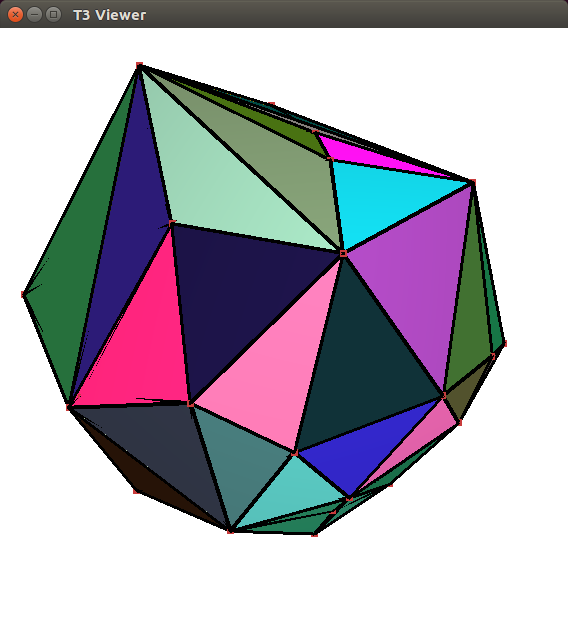Figure 45.6 Result of the run of the draw_triangulation_3 program. A window shows the 3D triangulation and allows to navigate through the 3D scene.

# Complexity and Performance

In 3D, the worst case complexity of a triangulation is quadratic in the number of points. For Delaunay triangulations, this bound is reached in cases such as points equally distributed on two non-coplanar lines. However, the good news is that, in many cases, the complexity of a Delaunay triangulation is linear or close to linear in the number of points. Several articles , , , ,  have proved such good complexity bounds for specific point distributions, such as points distributed on surfaces under some conditions.

## Running Time

There are several algorithms provided in this package. We will focus here on the following ones and give practical numbers on their efficiency :

• construction of a triangulation from a range of points,
• location of a point (using the locate function),
• removal of a vertex (using the remove function).

We will use the following types of triangulations, using Exact_predicates_inexact_constructions_kernel as geometric traits:

• Delaunay : Delaunay_triangulation_3
• Delaunay - Fast location : Delaunay_triangulation_3 with Fast_location
• Regular : Regular_triangulation_3 (default setting : memorize hidden points)
• Regular - No hidden points : Regular_triangulation_3 with hidden points discarded.

Figure 45.7 shows, for all these types of triangulations, the times in seconds taken to build a triangulation from a given number of points, then the average time to perform one point location in triangulations of various sizes, and the average time to perform one vertex removal (which is largely independent on the size of the triangulation).

The data sets used here are points randomly distributed in the unit cube (the coordinates are generated using the drand48 C function). In the weighted case, the weights are all zero, which means that there are actually no hidden points during execution.

The measurements have been performed using CGAL 3.6, using the GNU C++ compiler version 4.3.2, under Linux (Fedora 10 distribution), with the compilation options -O3 -DCGAL_NDEBUG. The computer used was equipped with a 64bit Intel Xeon 3GHz processor and 32GB of RAM (a recent desktop machine as of 2009).

 Delaunay Delaunay Regular Regular Fast location No hidden points Construction from $$10^2$$ points 0.00054 0.000576 0.000948 0.000955 Construction from $$10^3$$ points 0.00724 0.00748 0.0114 0.0111 Construction from $$10^4$$ points 0.0785 0.0838 0.122 0.117 Construction from $$10^5$$ points 0.827 0.878 1.25 1.19 Construction from $$10^6$$ points 8.5 9.07 12.6 12.2 Construction from $$10^7$$ points 87.4 92.5 129 125 Point location in $$10^2$$ points 9.93e-07 1.06e-06 7.19e-06 6.99e-06 Point location in $$10^3$$ points 2.25e-06 1.93e-06 1.73e-05 1.76e-05 Point location in $$10^4$$ points 4.79e-06 3.09e-06 3.96e-05 3.76e-05 Point location in $$10^5$$ points 2.98e-05 6.12e-06 1.06e-04 1.06e-04 Point location in $$10^6$$ points 1e-04 9.65e-06 2.7e-04 2.67e-04 Point location in $$10^7$$ points 2.59e-04 1.33e-05 6.25e-04 6.25e-04 Vertex removal 1e-04 1.03e-04 1.42e-04 1.38e-04

Figure 45.7 Running times in seconds for algorithms on 3D triangulations.

More benchmarks comparing CGAL to other software can be found in .

## Parallel Performance

Figure Figure 45.8 shows insertion and removal speed-ups obtained using the parallel version of the triangulation algorithms of CGAL 4.5. The machine used is a PC running Windows 7 64-bits with two 6-core Intel Xeon CPU X5660 clocked at 2.80 GHz with 32GB of RAM. The program has been compiled with Microsoft Visual C++ 2012 in Release mode.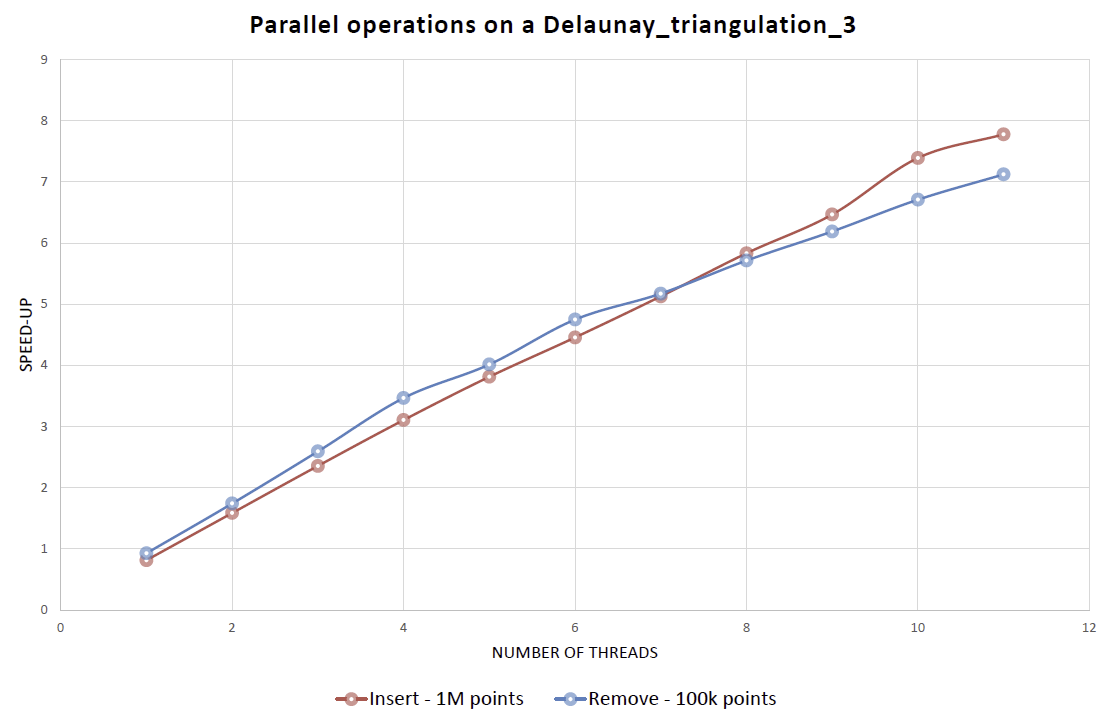Figure 45.8 Speed-up obtained for the insertion of 1M points randomly generated inside a cube (red), and the removal of 100K of them (blue), compared to the sequential version of the algorithm.

## Memory Usage

We give here some indication about the memory usage of the triangulations. Those structures being intensively based on pointers, the size almost doubles on 64bit platforms compared to 32bit.

The size also depends on the size of the point type which is copied in the vertices (hence on the kernel). Obviously, any user data added to vertices and cells also affect the memory used.

More specifically, the memory space used to store a triangulation is first a function of the size of its Vertex and Cell types times their numbers (and for volumic distribution, one sees about 6.7 times more cells than vertices). However, these are stored in memory using Compact_container, which allocates them in lists of blocks of growing size, and this requires some additional overhead for bookkeeping. Moreover, memory is only released to the system when clearing or destroying the triangulation. This can be important for algorithms like simplifications of data sets which will produce fragmented memory usage (doing fresh copies of the data structures are one way out in such cases). The asymptotic memory overhead of Compact_container for its internal bookkeeping is otherwise on the order of $$O(\sqrt{n})$$.

Figure 45.9 shows the number of bytes used per points, as measured empirically using Memory_sizer for large triangulations ( $$10^6$$ random points).

 Delaunay Delaunay Regular Regular Fast location No hidden points 32bit 274 291 336 282 64bit 519 553 635 527

Figure 45.9 Memory usage in bytes per point for large data sets.

## Variability Depending on the Data Sets and the Kernel

Besides the complexity of the Delaunay triangulation that varies with the distribution of the points, another critical aspect affects the efficiency : the degeneracy of the data sets. These algorithms are quite sensitive to numerical accuracy and it is important to run them using exact predicates.

Using a kernel with no exact predicates will quickly lead to crashes or infinite loops once they are executed on non-random data sets. More precisely, problems appear with data sets which contain (nearly) degenerate cases for the orientation and side_of_oriented_sphere predicates, namely when there are (nearly) coplanar or (nearly) cospherical points. This unfortunately happens often in practice with data coming from various kinds of scanners or other automatic acquisition devices.

Using an inexact kernel such as Simple_cartesian<double> would lead to optimal performance, which is only about 30% better than Exact_predicates_inexact_constructions_kernel. The latter is strongly recommended since it takes care about potential robustness issues. The former can be used for benchmarking purposes mostly, or when you really know that your data sets won't exhibit any robustness issue.

Exact predicates take more time to compute when they hit (nearly) degenerate cases. Depending on the data set, this can have a visible impact on the overall performance of the algorithm or not.

Sometimes you need exact constructions as well, so Exact_predicates_exact_constructions_kernel is a must. This is the case for example when you need the dual functions to be exact, or when your input is stored in points of such a kernel for other reasons (because it is the output of another algorithm which has this requirement, for example). This will slow down the computations by a factor of 4 to 5 at least, and it can be much more.

Figure 45.10 gives more detailed timings about various kernels one the following data sets : random points in a cube, random points on the surface of an ellipsoid, points scanned on the surface of a Buddha statue, points on a molecular surface, and points scanned on a dryer handle. See Figure 45.11 for pictures of the last 3 objects, which respectively illustrate volumic data, surfacic data, and data with many degenerate cases. This last data set exhibits an infinite loop with an inexact kernel, and of course we are not sure whether what is computed for the other data sets with this inexact kernel is a Delaunay triangulation. General introductory information about these robustness issues can be found in . More benchmarks around this issue can also be found in .

 Random Ellipsoid Buddha Molecule Dryer Handle Number of points 100000 100000 542548 525296 49787 Simple_cartesian 0.69 0.627 4.21 3.8 $$\infty$$-loop Exact_predicates_inexact_constructions_kernel 0.824 0.749 4.99 4.64 1.68 Exact_predicates_exact_constructions_kernel 4.59 3.85 30.1 26.4 4.57 Simple_cartesian 492 534 1120 1030 75.2

Figure 45.10 Running times (seconds) for various kernels and data sets.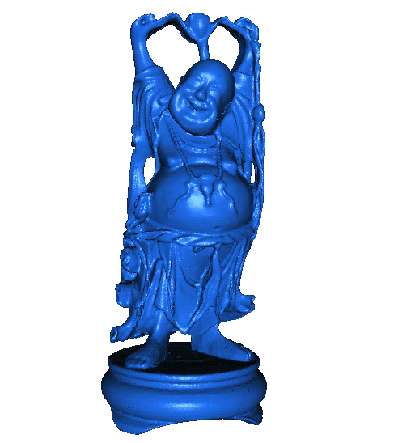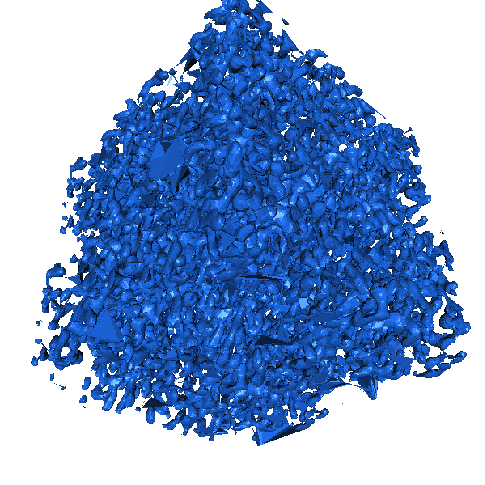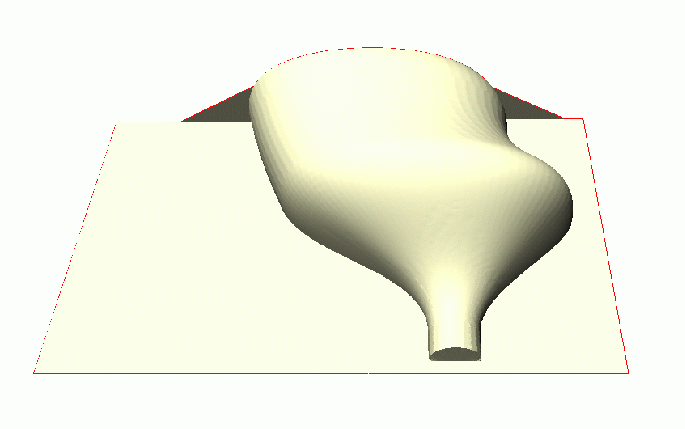Figure 45.11 Data sets used in the benchmark of Figure 45.10.

# Design and Implementation History

Monique Teillaud started to work on the 3D triangulation packages in 1997, following the design of the 2D triangulation packages. The notions of degenerate dimensions and infinite vertex were formalized  and induced changes in the 2D triangulation packages. The packages were first released in CGAL 2.1. They contained basic functionalities on triangulations, Delaunay triangulations, regular triangulations.

A first version of removal of a vertex from a Delaunay triangulation was released in CGAL 2.2. However, this removal became really robust only in CGAL 2.3, after some research that allowed to deal with degenerate cases quite easily . Andreas Fabri implemented this revised version of the removal, and a faster removal algorithm for CGAL 3.0.

The latter algorithm was proposed by Mariette Yvinec, who contributed in several ways to the package, first since she was maintaining the close 2D triangulation package and participated in many discussions, she also wrote the traits classes for regular triangulations.

In 2000, Sylvain Pion started working on these packages. He improved the efficiency of triangulations in CGAL 2.3 and 2.4 in several ways  he implemented the Delaunay hierarchy  in 2.3, he improved the memory footprint in 2.4 and 3.0, he also performed work on arithmetic filters  (see Filtered_kernel) to improve the speed of triangulations. He changed the design in CGAL 3.0, allowing users to add handles in their own vertices and cells.

Olivier Devillers, co-author of preliminary versions of the CGAL 2d triangulations, participated in many discussions, in particular about the perturbations, and more concretely in the implementation of the Delaunay hierarchy.

In 2005, Christophe Delage implemented the vertex removal function for regular triangulations, using the symbolic perturbation proposed in , which allowed to release this functionality in CGAL 3.2.

In 2006, Nico Kruithof wrote the Triangulation_simplex_3 class that can store simplices of any dimension and improved the internal organization of the code.

As of March 2007, Christophe Delage made the iterator range insert methods and constructors use spatial_sort to improve efficiency.

In 2008, Camille Wormser added a few more iterators in the package that were integrated in release 3.4.

In 2009, Sylvain Pion simplified the design of the Delaunay hierarchy so that it became the simple Fast_location policy in release 3.6.

In 2010, Pedro de Castro and Olivier Devillers added the point displacement in release 3.7.

In 2011, Pedro de Castro and Olivier Devillers implemented in release 3.8 the structural filtering method, improving the efficiency of point location.

A new demo of this package was introduced in CGAL 3.8, coded by Fei (Sophie) Che, who was co-mentored by Manuel Caroli and Monique Teillaud in the framework of the Google Summer of Code, 2010.

In 2013, Clément Jamin added parallel algorithms (insert, remove) to the Delaunay and regular triangulations.

In 2014, Thijs van Lankveld implemented the segment cell traverser iterator. In 2018, Jane Tournois introduced the segment simplex traverser iterator and finalized both iterators that were integrated in release 5.2. Olivier Devillers contributed to boost the performances of the cell traverser.

The authors wish to thank Lutz Kettner for inspiring discussions about the design of CGAL. Jean-Daniel Boissonnat is also acknowledged .